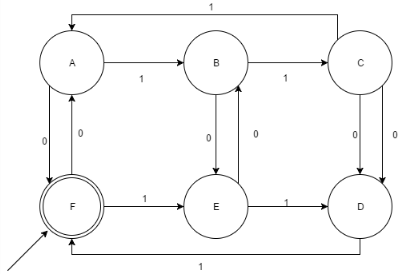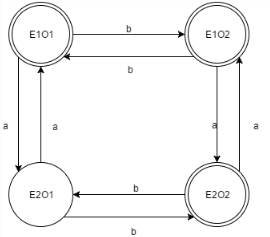Test: The Language of DFA

# Test: The Language of DFA - Computer Science Engineering (CSE)

Test Description

## 10 Questions MCQ Test Theory of Computation - Test: The Language of DFA

Test: The Language of DFA for Computer Science Engineering (CSE) 2023 is part of Theory of Computation preparation. The Test: The Language of DFA questions and answers have been prepared according to the Computer Science Engineering (CSE) exam syllabus.The Test: The Language of DFA MCQs are made for Computer Science Engineering (CSE) 2023 Exam. Find important definitions, questions, notes, meanings, examples, exercises, MCQs and online tests for Test: The Language of DFA below.
Solutions of Test: The Language of DFA questions in English are available as part of our Theory of Computation for Computer Science Engineering (CSE) & Test: The Language of DFA solutions in Hindi for Theory of Computation course. Download more important topics, notes, lectures and mock test series for Computer Science Engineering (CSE) Exam by signing up for free. Attempt Test: The Language of DFA | 10 questions in 10 minutes | Mock test for Computer Science Engineering (CSE) preparation | Free important questions MCQ to study Theory of Computation for Computer Science Engineering (CSE) Exam | Download free PDF with solutions
 1 Crore+ students have signed up on EduRev. Have you?
Test: The Language of DFA - Question 1

### How many languages are over the alphabet R?

Detailed Solution for Test: The Language of DFA - Question 1

A language over an alphabet R is a set of strings over A which is uncountable and infinite.

Test: The Language of DFA - Question 2

### According to the 5-tuple representation i.e. FA= {Q, ∑, δ, q, F}Statement 1: q ϵ Q’; Statement 2: FϵQ

Detailed Solution for Test: The Language of DFA - Question 2

Q is the Finite set of states, whose elements i.e. the states constitute the finite automata.

Test: The Language of DFA - Question 3

### δˆ tells us the best:

Detailed Solution for Test: The Language of DFA - Question 3

δ or the Transition function describes the best, how a DFA behaves on a string where to transit next, which direction to take.

Test: The Language of DFA - Question 4

Which of the following option is correct?A= {{abc, aaba}. {ε, a, bb}}

Detailed Solution for Test: The Language of DFA - Question 4

As the question has dot operation, ε will not be a part of the concatenated set. Had it been a union operation, ε would be a part of the operated set.

Test: The Language of DFA - Question 5

For a DFA accepting binary numbers whose decimal equivalent is divisible by 4, what are all the possible remainders?

Detailed Solution for Test: The Language of DFA - Question 5

All the decimal numbers on division would lead to only 4 remainders i.e. 0,1,2,3 (Property of Decimal division).

Test: The Language of DFA - Question 6

Which of the following x is accepted by the given DFA (x is a binary string ∑= {0,1})?Detailed Solution for Test: The Language of DFA - Question 6

Explanation : The given DFA accepts all the binary strings such that they are divisible by 3 and 2.Thus, it can be said that it also accepts all the strings which is divisible by 6.

Test: The Language of DFA - Question 7

Given:
L1= {xϵ ∑*|x contains even no’s of 0’s}
L2= {xϵ ∑*|x contains odd no’s of 1’s}
No of final states in Language L1 U L2?

Detailed Solution for Test: The Language of DFA - Question 7Test: The Language of DFA - Question 8

The maximum number of transition which can be performed over a state in a DFA?∑= {a, b, c}

Detailed Solution for Test: The Language of DFA - Question 8

The maximum number of transitions which a DFA allows for a language is the number of elements the transitions constitute.

Test: The Language of DFA - Question 9

The maximum sum of in degree and out degree over a state in a DFA can be determined as:

∑= {a, b, c, d}

Detailed Solution for Test: The Language of DFA - Question 9

The out degree for a DFA I fixed while the in degree depends on the number of states in the DFA and that cannot be determined without the dependence over the Language.

Test: The Language of DFA - Question 10

The sum of minimum and maximum number of final states for a DFA n states is equal to:

Detailed Solution for Test: The Language of DFA - Question 10

The maximum number of final states for a DFA can be total number of states itself and minimum would always be 1, as no DFA exits without a final state. Therefore, the solution is n+1.

## Theory of Computation

18 videos|56 docs|44 tests
Information about Test: The Language of DFA Page
In this test you can find the Exam questions for Test: The Language of DFA solved & explained in the simplest way possible. Besides giving Questions and answers for Test: The Language of DFA, EduRev gives you an ample number of Online tests for practice

## Theory of Computation

18 videos|56 docs|44 tests